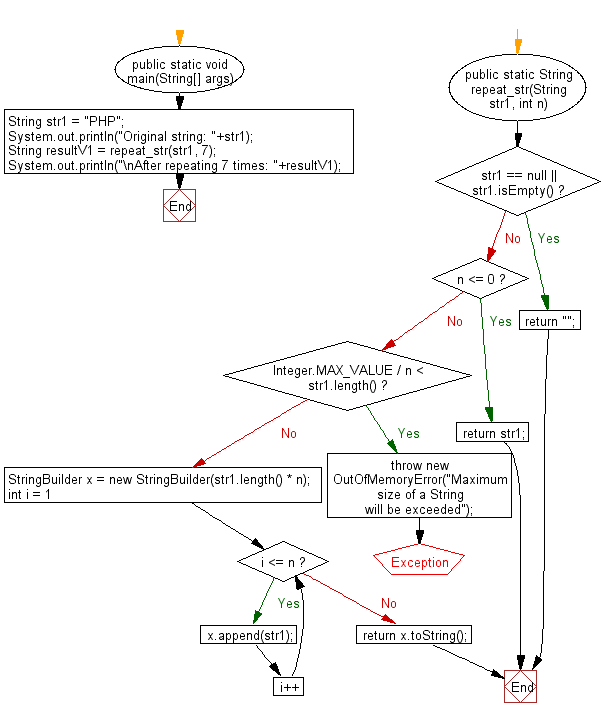﻿ Java - Concatenate a string with itself of a number of times

# Java: Concatenate a given string with itself of a given number of times

## Java String: Exercise-106 with Solution

Write a Java program to concatenate a given string with itself a given number of times.

Visual Presentation:Sample Solution:

Java Code:

``````import java.util.Arrays;

public class Main {
public static void main(String[] args) {
String str1 = "PHP"; // Declare and initialize a string variable
System.out.println("Original string: " + str1); // Display the original string
String resultV1 = repeat_str(str1, 7); // Call the repeat_str method with str1 and 7 as arguments
System.out.println("\nAfter repeating 7 times: " + resultV1); // Display the result of repeating str1 7 times
}

public static String repeat_str(String str1, int n) {
// Check if the input string is null or empty
if (str1 == null || str1.isEmpty()) {
return ""; // Return an empty string
}

// Check if n is less than or equal to 0
if (n <= 0) {
return str1; // Return the original string as n is 0 or negative
}

// Check if multiplying the length of str1 by n will exceed the maximum size of a String
if (Integer.MAX_VALUE / n < str1.length()) {
throw new OutOfMemoryError("Maximum size of a String will be exceeded"); // Throw an error
}

StringBuilder x = new StringBuilder(str1.length() * n); // Create a StringBuilder to store the result
// Repeat the string n times and append it to the StringBuilder
for (int i = 1; i <= n; i++) {
x.append(str1);
}
return x.toString(); // Return the final repeated string
}
}
``````

Sample Output:

```Original string: PHP

After repeating 7 times: PHPPHPPHPPHPPHPPHPPHP
```

Flowchart:Java Code Editor:

Improve this sample solution and post your code through Disqus

What is the difficulty level of this exercise?

Test your Programming skills with w3resource's quiz.

﻿